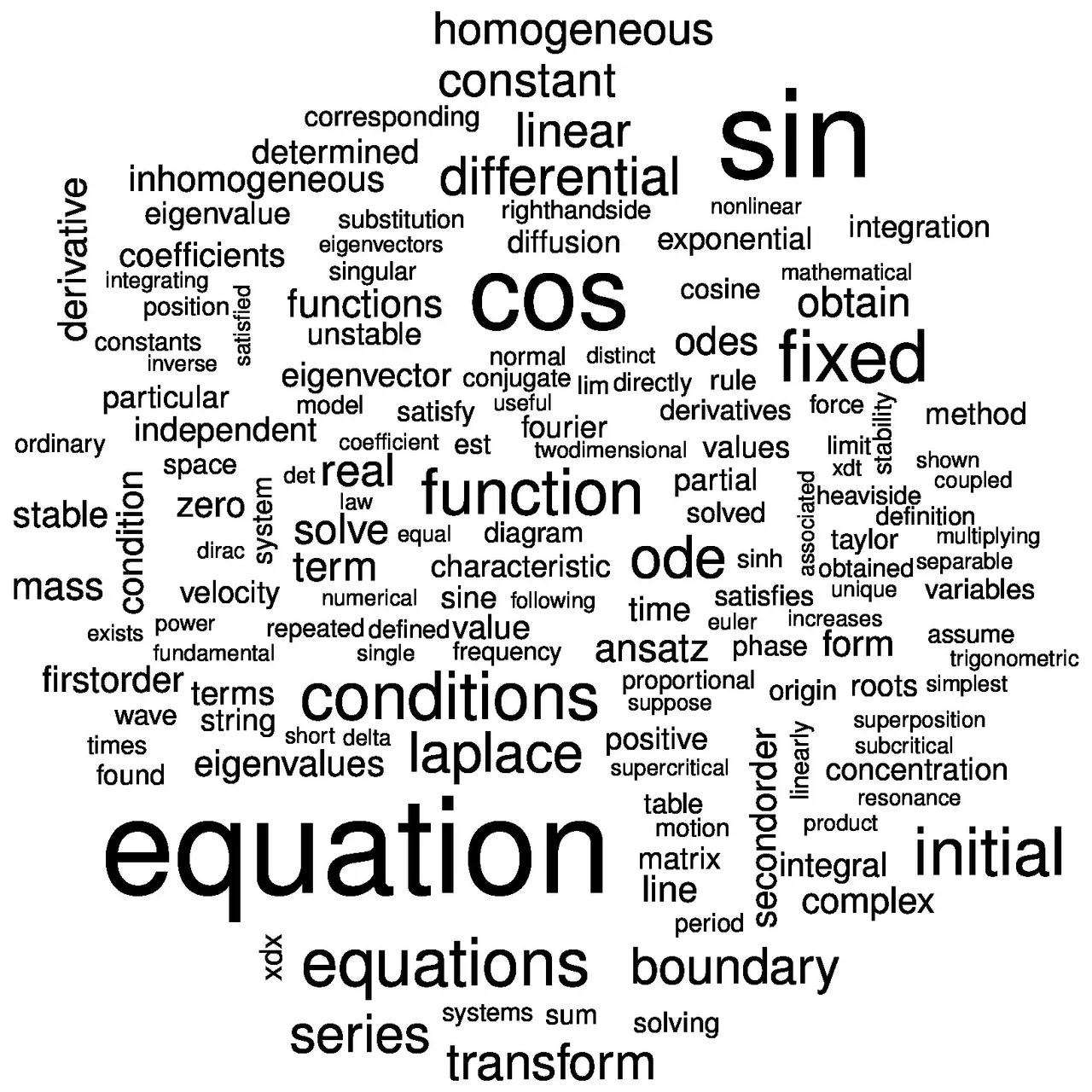# Mathematics - Differential equations IntroductionHello it's a me again drifter1! Today we get back to my math series to start talking about Differential equations! This post is an introduction and we will get into some theory and the applications of differential equations. So, without further do, let's get started!

## What you need to know

To understand and solve differential equations you must need to know differentiation and integration. I covered all those things and more in my Mathematical Analysis subseries of mathematics.

The posts that I would suggest you to check are:

Derivatives

Integrals

And for some of the solutions you might also want to know integration techniques that depend on the type of integral that we end up with in our examples. We will need simple integrals in most of the example-cases, so don't be worried if you don't know that that good! :)

## Definition

So, what is an differential equation?

An mathematical equation that contains an unknown function with its derivatives.

The equations that contain only one variable x are called ordinary differential equations, and those are the ones that we will cover in my series for sake of simplicity!

### Form

So, the general form of an ordinary differential equation (ODE) is:

F(x, y, y', y'', ..., y^(n)) = 0

where y^(n) is the n-th derivative of y or f(x).

An example could be:
x^3 + y + 5y' - 2y''' = 0

or even y'' = 0

### Order

The natural number n of the biggest-order derivative in the D.E. is called order of the D.E.

For example the 1st DE of the previous example has an order of 3, cause the term 2y''' has the biggest derivative y''', which is the 3rd derivative of y = f(x).

This seperation into orders and types will help us when solving them!

### SolutionAn function y = f(x) is an (particular) solution of an DE when it verifies it, which means that:

F(x, f(x), f'(x), ..., f^(n)(x)) = 0

This solution may not be unique!

The general solution of an DE is an function y = f(x, c1, c2, ..., cn) that also verifies the DE, but for the different values of c1, c2, ..., cn in R.

This means that solutions that don't come from the general solution are singular solutions.

(they mostly occur in non-linear equations)

For some of the DE types we have solving methods. We will get into how we solve the most useful and essential of them!

### Applications

So finally, let's talk about why we need them and how we use them.Differential equations are used in physics, engineering and more and can help when calculating the rate of change of specific variables/physical quantities.

For example Newton's second law can be applied with an non-constant force and so acceleration.

F = ma becomes F(t) = m * a(t) = m * u'(t) = m * s"(t) =>

F(t) = m * s"(t)

(That we talked about in my Physics series)

We can see that the value of the force in time is also an differential equation m * s"(t), where we must find the second-derivative of the distance/displacement.

We could also do the opposite, when knowing the force in time, to find the displacement (s) and so velocity (u), acceleration (a) by differentiating it.

You can clearly see that DE's are very useful in many different science branches, because of the generalizability of mathematics. They are not so difficult to understand and solve, and that's why they are prefered over other solution methods of problems with physical and non-physical quantities.

### Image sources:And this is actually it for today and I hope that you enjoyed it!

Next time we will get into the types of differential equations and how we solve some of the first-order type ones!

See ya!

H2
H3
H4
3 columns
2 columns
1 column